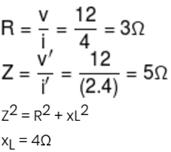Courses

# Physics Test 8 - Electrostatics, Capacitance, Current Electricity, Magnetic Effect, Magnetism, Emi And AC

## 25 Questions MCQ Test Mock Test Series for JEE Main & Advanced 2022 | Physics Test 8 - Electrostatics, Capacitance, Current Electricity, Magnetic Effect, Magnetism, Emi And AC

Description
This mock test of Physics Test 8 - Electrostatics, Capacitance, Current Electricity, Magnetic Effect, Magnetism, Emi And AC for JEE helps you for every JEE entrance exam. This contains 25 Multiple Choice Questions for JEE Physics Test 8 - Electrostatics, Capacitance, Current Electricity, Magnetic Effect, Magnetism, Emi And AC (mcq) to study with solutions a complete question bank. The solved questions answers in this Physics Test 8 - Electrostatics, Capacitance, Current Electricity, Magnetic Effect, Magnetism, Emi And AC quiz give you a good mix of easy questions and tough questions. JEE students definitely take this Physics Test 8 - Electrostatics, Capacitance, Current Electricity, Magnetic Effect, Magnetism, Emi And AC exercise for a better result in the exam. You can find other Physics Test 8 - Electrostatics, Capacitance, Current Electricity, Magnetic Effect, Magnetism, Emi And AC extra questions, long questions & short questions for JEE on EduRev as well by searching above.
QUESTION: 1

Solution:
QUESTION: 2

### Work done in carrying a charge ‘Q’ from ‘A’ to ‘B’ (as shown in figure) on a circle of radius ‘r’ with a charge ‘Q’ at the centre is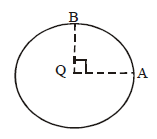Solution: A circle around a point charge represents an equipotential surface (V= Kq/r throughout the perimeter), and work done on an equipotential surface is zero.
QUESTION: 3

### Two spheres having radii of 5 cm and 10 cm bear identical charge of 6.6 nC. Find the potential of spheres after they have been connected by a conductor, assume that the spheres are at large distance from each other

Solution:
QUESTION: 4

An ideal electrical cell does 5 joule of work in carrying 10 coulomb of charge around a closed circuit. The heat loss or energy consumption in the circuit outside the battery is

Solution:
QUESTION: 5

A charged particle of mass 0.1 g is in equilibrium in an electric field E = 98 N/C. The charge on the particle is

Solution:
QUESTION: 6

An electric charge ‘q’ is lying at the open end of a cylindrical vessel. The flux coming out of the cylinder will be

Solution: If you put a another cylinder on it then charge will completely in side and flux will distribute half half so flux =q/2εₒ
QUESTION: 7

On moving a charge of 20 coulombs by 2cm, 2J of work is done, then the potential difference between the points is

Solution: Va - Vb =W/q.
W= work done :2Jq= charge : 20C Va - Vb = 2/20 = 0.1
QUESTION: 8

The capacitance of a parallel plate condenser does not depend upon

Solution:
QUESTION: 9

A semi-circular are of radius ‘a’ is charged uniformly and the charge per unit length is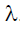. The electric field at its centre is

Solution:
QUESTION: 10

Which of the following cannot be the units of electric intensity?

Solution:
QUESTION: 11

The unit of permittivity of free space ∈0  is

Solution:

Solution :- By coulomb's law the electrostatic force

F = 1/4πε0(q1q2/r2)

⇒ε0 = 1/4πF(q1q2/r2)

Substituting the unit for q, r,and F , we abtain unit of

ε0 = coulomb×coulomb newton−(metre)2

= (coulumb)2 newton −(meter)2

=C2N−m2

QUESTION: 12

Ampere hour is a unit of

Solution:
QUESTION: 13

Six resistors each of value 10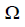, are connected to a 5 V battery as shown in the figure. The reading of ammeter'A' is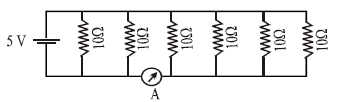Solution:
QUESTION: 14

The potential difference between points ‘A’ and ‘B(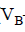-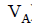)' in the figure if R=0.7is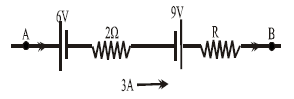Solution: Ans) Applying Kirchhoff's first law
Va - 6 - 2(3) + 9 - (0.7)(3) =Vb
Vb - Va = 9- 6 - 6 - 2.1
=- 3 - 2.1
= - 5.1
QUESTION: 15

If 2% of the main current is to be passed through the galvanometer of resistance G. the resistance of shunt required is

Solution:
Let total current through the circuit be I.
Then, 0.02I current is to be passed through galvanometer of resistance G.
Let the resistance of shunt is Rs .
∵ Shunt are in parallel combination with Galvanometer.
∴ 0.02I * G = (1 - 0.02)I * Rs
⇒0.02G = 0.98 * Rs
⇒Rs = 0.02G/0.98 = G/49

QUESTION: 16

The thermo e.m.f. of a thermocouple is given by e = 2164 t - 6.2 t2 . The neutral temperature and a temperature of inversion are

Solution:
QUESTION: 17

The relation between Faraday constant F, electron charge e, and Avogadro's Number N is

Solution:
QUESTION: 18

In the circuit as shown in Fig. The heat produced by 6resistance due to current flowing in it is 60 calories per second. The heat generated across 3 ohm resistance per second will beSolution:
QUESTION: 19

A thin bar magnet of length 2l and breadth 2b, pole strength p and magnetic moment M is divided into four equal parts with length and breadth of each part being half of the original magnet. Then the pole strength of each part is

Solution:
QUESTION: 20

Susceptibility is positive and small for a ........substance:

Solution:
*Answer can only contain numeric values
QUESTION: 21

A battery of internal resistance 4 ohm is connected to the network of resistance as shown. In the order that the maximum power can be delivered to the network, the value of R in ohm should be :-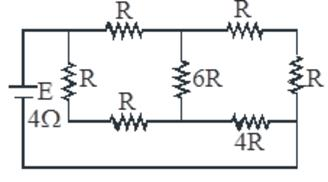Solution:

Condition for maximum power is
r = R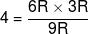R = 2

*Answer can only contain numeric values
QUESTION: 22

A capacitor of capacitance 5 μF is connected as shown in the fig. The internal resistance of the cell is 0.5 Ω. The amount of charge on the capacitor plate is :- (μC) :-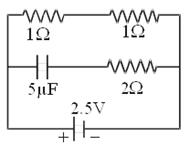Solution:
*Answer can only contain numeric values
QUESTION: 23

Consider two concentric non-conducting uniformly charged spherical shells of radius 3m and 6m having charges 10 µC and 200 µC respectively. Find the interaction potential energy between two spheres in joules.

Solution: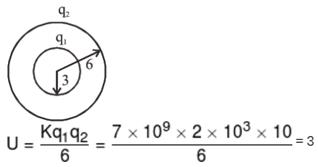*Answer can only contain numeric values
QUESTION: 24

Diagram shows a part of circuit. Find VA–VB when current I is 5A and it is decreasing at a rate of 10+3 A/s ?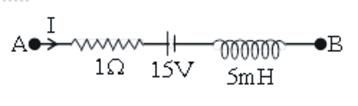Solution: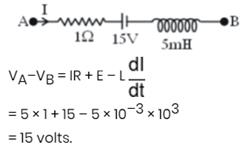*Answer can only contain numeric values
QUESTION: 25

A current of 4 A flows in a coil when connected to a 12 V d.c. source. If the same coil is connected to a 12 V, 50 rad/s, a.c. source, a current of 2.4 A flows in the circuit. Determine the reactance (in Ω) of coil.

Solution: